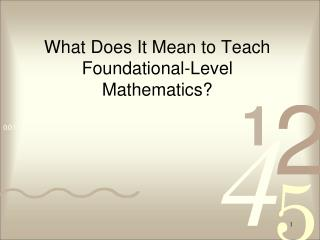# What Does It Mean to Teach Foundational-Level Mathematics? - PowerPoint PPT PresentationDownload PresentationWhat Does It Mean to Teach Foundational-Level Mathematics?

What Does It Mean to Teach Foundational-Level Mathematics?
Download Presentation## What Does It Mean to Teach Foundational-Level Mathematics?

- - - - - - - - - - - - - - - - - - - - - - - - - - - E N D - - - - - - - - - - - - - - - - - - - - - - - - - - -
##### Presentation Transcript

1. What Does It Mean to Teach Foundational-Level Mathematics?

2. Warm Up Problem – Try This! Shade in six (6) squares in the given rectangle. Using the figure, determine the percent of the area that is shaded in at least two ways. Your reasoning should make sense in relation to the figure, not simply consist of numerical calculations! Discuss with a partner the strategies you used and why they work. Relate your strategies to the figure. Source: Stein, Smith, Henningsen, & Silver (2000).Implementing Standards-Based Mathematics Instruction. New York: Teachers College Press

3. Sample Responses Since there are 10 rows, each row is 10%. 6 squares give me 1 ½ rows, so that is 10% + 5% = 15%. Take away the bottom row – that’s 10%. The remaining 90% can be cut into 6 congruent rectangles like the shaded one. So, six squares is 90/6 = 15%. There are 40 squares in the original. I know percent is out of 100, so I can add 40 more squares then 20 more squares to get 100. Since 40 * 2 ½ is 100, then 6 * 2 ½ = 15%.

4. Why the FLM Credential? • Created by CA in 2003. • NCLB compliance, especially middle grades. • Aimed at those with a strong mathematics background but not necessarily a math major. • “Foundational-Level Mathematics” connotes the idea that content preceding algebra and continuing through geometry forms the foundation for higher level coursework in mathematics. • Allows teaching in general mathematics, algebra, geometry, probability and statistics, and consumer mathematics. No AP courses can be taught.

5. Why the FLM Credential? More than 80% of mathematics classes in grades 7-12 can be taught by FLM teachers in addition to any math in grades K-6.

6. What is Required for Earning an FLM Teaching Credential? • At least a Bachelor’s degree (prefer math-based major) • Passing score on CSET Mathematics I and II Exams • Suggested coursework in mathematics: • Algebra, Trigonometry, Pre-Calculus • Calculus (1 semester) • Probability and Statistics • Math for Teachers courses • Education coursework: • Methods of Teaching • Adolescent Development • Teaching English Learners • Diversity and Schooling • Teaching Literacy • Using Technology in Teaching • NOTE: If you are Multiple Subject credentialed, you may earn FLM certification by passing the CSET requirements and taking a secondary mathematics methods course.

7. CSET Exams in Mathematics • Exam I and II required for FLM eligibility • Exam I: Algebra and Number Theory • Exam II: Geometry and Probability & Statistics • CSET website with list of content and sample items: http://www.cset.nesinc.com/CS_testguide_Mathopener.asp • Orange County Department of Education (OCDE) offers a CSET Mathematics Preparation course. Call 714-966-4156. • Website of a mathematics teacher in Riverside who has passed all of the CSET Mathematics exams: http://innovationguy.easyjournal.com/

8. What Does It Mean to Teach Mathematics to ALL Students? • What percentage of California 8th graders take algebra? • 1996: 25% • 2003: 45% • The pass rate for Algebra I, historically, has been about 50-60%. • How can we meet the needs of all students, particularly those whose needs have not been well-served by “traditional” education practices?

9. Bridging from Number Operations to Algebraic Thinking • Pre-K to 5 mathematics develops: • Number sense within the Base 10 system • Procedural fluency with whole number operations (+, –, x, ÷) • Concept of rational number • Concrete methods of mathematical reasoning • Grade 6 – 8 mathematics develops: • Number sense with rational numbers • Procedural fluency with rational number operations • Movement from additive to multiplicative comparisons • Communication skills in math, written and oral • Reasoning and problem solving skills • Abstract models of mathematical reasoning (algebra)

10. Mathematical Proficiency • Adding It Up: Helping Children Learn Mathematics, NRC (2001) • Must get beyond skills only focus and work toward developing reasoning and understanding in order to cultivate a productive disposition. • Proficiency is defined in terms of five interwoven strands.

11. Strands of Mathematical Proficiency • Conceptual understanding - comprehension of mathematical concepts, operations, and relations • Procedural fluency - skill in carrying out procedures flexibly, accurately, efficiently, and appropriately • Strategic competence - ability to formulate, represent, and solve mathematical problems

12. Strands of Mathematical Proficiency(cont’d) • Adaptive reasoning- capacity for logical thought, reflection, explanation, and justification • Productive disposition - habitual inclination to see mathematics as sensible, useful, and worthwhile, coupled with a belief in diligence and one’s own efficacy

13. Teaching Foundational-Level Mathematics • Focus on relationships, connections • Allow for and support student communication and interaction • Use multiple representations of mathematical concepts and relationships • Use contextualized and non-routine problems • Explicitly bridge student thinking from concrete to abstract